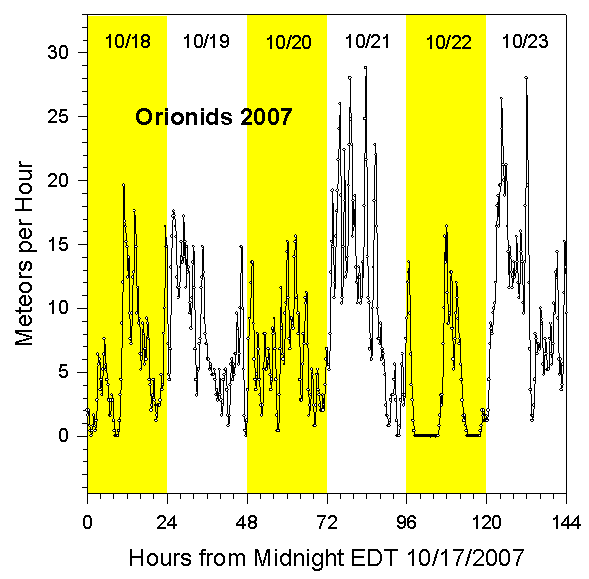Radio Observation of the 2007 Orionids

Data Collection and Analysis

For details about our equipment and method of data analysis, see our main page. The peak counting rate was about 29 meteors per hour during the day on October 21, 2007. The peak occurred at the predicted time, it was very broad, and the counting rate was as expected. There was considerable activity in the surrounding days. The meteors of this shower are from Halley's Comet.

In the following summary graph, we've counted meteors in quarter hour intervals, and then applied a data smoothing equation of the form

Smoothed_Rate(t) = 3.2*[((1/2)*N(t))+((1/4)*(N(t+1))+((1/4)*(N(t-1))+((1/8)*(N(t+2))+((1/8)*(N(t-2))]

where N(t) is the number of meteors in the fifteen minute interval t, N(t-1)is the number of meteors in the preceding fifteen minute interval, and so on.

Rates for 2007 Orionids

The following graph shows the meteor count rates per hour we measured for the 2007 Orionids. These rates were determined by a computer program that counted all peaks that climbed at least 20% above the background level in one second. The graph shows the rates observed for the six day interval starting at Midnight EDT on 10/17/07.Summary

The 2007 Orionid meteor shower had a peak counting rate was about 29 meteors per hour in a very broad peak during the day on October 21, 2007. There was considerable activity in the surrounding days. The peak occurred at the expected time, and the counting rate was about what was expected, based on observations in previous years.